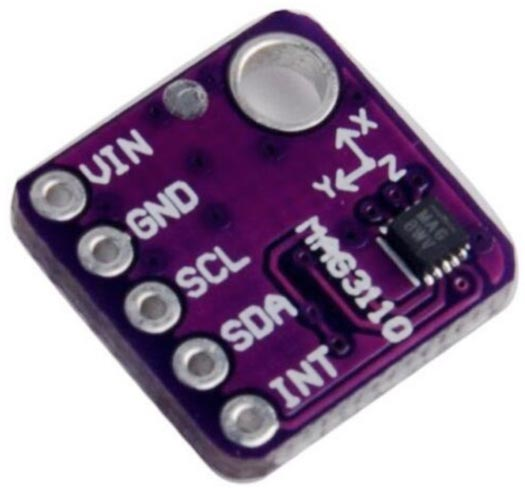## MAG3110 3-Axis Magnetometer

The NXP MAG3110  is a 3-axis digital magnetometer or digital compass. It has a 1.95 V to 3.6 V voltage range.

It is available on a breakout from AliExpress :The I2C address is #x0E or 14.

#### Initialising the sensor

The following routine enables automatic magnetic sensor resets, and puts the MAG3110 in active mode 80 Hz Output Data Rate with Over Sample Ratio = 1:

```(defun ini ()
(let (res)
(with-i2c (s 14)
(write-byte #x11 s) ; CTRL_REG2
(write-byte #x80 s) ; Automatic magnetic sensor resets
(restart-i2c s)
(write-byte #x10 s) ; CTRL_REG1
(write-byte #x01 s))))```

#### Reading the magnetometer

The following routine s16 returns a signed 16-bit integer from two bytes, MSB first:

```(defun s16 (s)
(let ((d (logior (ash (read-byte s) 8) (read-byte s))))
(- d (ash (logand d #x8000) 1))))```

Here's the routine xyz to read the magnetometer:

```(defun xyz ()
; Wait until new data ready
(loop
(with-i2c (s 14)
(write-byte 0 s) ; Status register
(restart-i2c s)
(when (plusp (read-byte s)) (return))))
(let (res)
(with-i2c (s 14)
(write-byte 1 s) ; X register
(restart-i2c s 6)
(dotimes (i 3)
(push (s16 s) res)))
(reverse res)))```

It returns a list of three integers for the three axes, in the order (x y z). For example:

```> (xyz)
(980 -904 -2704)```

#### Reading the temperature

The MAG3110 will also provide a temperature reading:

```(defun tmp ()
(with-i2c (s 14)
(write-byte #x0F s) ; Temp register
(restart-i2c s 1)
(let ((d (read-byte s)))
(- (logand d #x7F) (logand d #x80) -10))))```

The temperature offset needs to be calibrated by the user; I found the value -10 gave good readings with the device I tested.

1. ^ MAG3110 Datasheet on NXP.
2. ^ GY MAG3110 3 axis magnetometer on AliExpress.